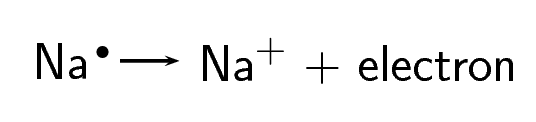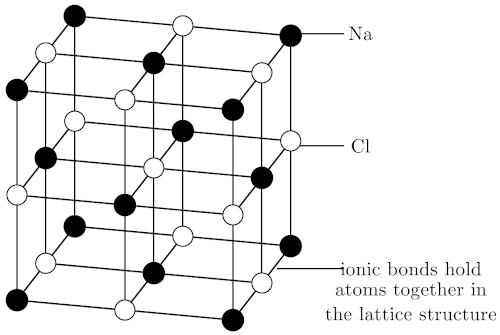Home Practice
For learners and parents For teachers and schools
Textbooks
Full catalogue
Pricing SupportLog in

We think you are located in United States. Is this correct?

# 6.4 Ionic bonding

## 6.4 Ionic bonding (ESABW)

### The nature of the ionic bond (ESABX)

When electrons are transferred from one atom to another it is called ionic bonding.

Electronegativity is a property of an atom, describing how strongly it attracts or holds onto electrons. Ionic bonding takes place when the difference in electronegativity between the two atoms is more than $$\text{1,7}$$. This usually happens when a metal atom bonds with a non-metal atom. When the difference in electronegativity is large, one atom will attract the shared electron pair much more strongly than the other, causing electrons to be transferred to the atom with higher electronegativity. When ionic bonds form, a metal donates one or more electrons, due to having a low electronegativity, to form a positive ion or cation. The non-metal atom has a high electronegativity, and therefore readily gains electrons to form a negative ion or anion. The two ions are then attracted to each other by electrostatic forces.

Ionic bond

An ionic bond is a type of chemical bond where one or more electrons are transferred from one atom to another.

Example 1:

In the case of $$\text{NaCl}$$, the difference in electronegativity between $$\text{Na}$$ ($$\text{0,93}$$) and $$\text{Cl}$$ ($$\text{3,16}$$) is $$\text{2,1}$$. Sodium has only one valence electron, while chlorine has seven. Because the electronegativity of chlorine is higher than the electronegativity of sodium, chlorine will attract the valence electron of the sodium atom very strongly. This electron from sodium is transferred to chlorine. Sodium loses an electron and forms an $$\text{Na}^{+}$$ ion.Chlorine gains an electron and forms a $$\text{Cl}^{-}$$ ion.Chlorine is a diatomic molecule and so for it to take part in ionic bonding, it must first break up into two atoms of chlorine. Sodium is part of a metallic lattice and the individual atoms must first break away from the lattice.

The electron is therefore transferred from sodium to chlorine:

The balanced equation for the reaction is:

$2\text{Na} + \text{Cl}_{2} \rightarrow 2\text{NaCl}$

Example 2:

Another example of ionic bonding takes place between magnesium $$(\text{Mg})$$ and oxygen $$(\text{O}_{2})$$ to form magnesium oxide $$(\text{MgO})$$. Magnesium has two valence electrons and an electronegativity of $$\text{1,31}$$, while oxygen has six valence electrons and an electronegativity of $$\text{3,44}$$. Since oxygen has a higher electronegativity, it attracts the two valence electrons from the magnesium atom and these electrons are transferred from the magnesium atom to the oxygen atom. Magnesium loses two electrons to form $$\text{Mg}^{2+}$$, and oxygen gains two electrons to form $$\text{O}^{2-}$$. The attractive force between the oppositely charged ions is what holds the compound together.

The balanced equation for the reaction is:

$2\text{Mg} + \text{O}_{2} \rightarrow 2\text{MgO}$

Because oxygen is a diatomic molecule, two magnesium atoms will be needed to combine with one oxygen molecule (which has two oxygen atoms) to produce two units of magnesium oxide $$(\text{MgO})$$.

temp text

### The crystal lattice structure of ionic compounds (ESABY)

Ionic substances are actually a combination of lots of ions bonded together into a giant molecule. The arrangement of ions in a regular, geometric structure is called a crystal lattice. So in fact $$\text{NaCl}$$ does not contain one $$\text{Na}$$ and one $$\text{Cl}$$ ion, but rather a lot of these two ions arranged in a crystal lattice where the ratio of $$\text{Na}$$ to $$\text{Cl}$$ ions is 1:1. The structure of the crystal lattice is shown below.Figure 6.2: The crystal lattice arrangement in $$\text{NaCl}$$Figure 6.3: A space filling model of the sodium chloride lattice

### Properties of ionic compounds (ESABZ)

Ionic compounds have a number of properties:

1. Ions are arranged in a lattice structure

2. Ionic solids are crystalline at room temperature

3. The ionic bond is a strong electrostatic attraction. This means that ionic compounds are often hard and have high melting and boiling points

4. Ionic compounds are brittle and bonds are broken along planes when the compound is put under pressure (stressed)

5. Solid crystals do not conduct electricity, but ionic solutions do

## Ionic compounds

Textbook Exercise 6.3

Explain the difference between a covalent and an ionic bond.

Solution not yet available.

Magnesium and chlorine react to form magnesium chloride.

1. What is the difference in electronegativity between these two elements?

2. Give the chemical formula for:

1. a magnesium ion

2. a chloride ion

3. the ionic compound that is produced during this reaction

3. Write a balanced chemical equation for the reaction that takes place.

Solution not yet available.

Draw Lewis diagrams to represent the following ionic compounds:

1. sodium iodide $$(\text{NaI})$$

2. calcium bromide $$(\text{CaBr}_{2})$$

3. potassium chloride $$(\text{KCl})$$

Solution not yet available.